In the parabola equation above,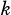is a constant. When the parabola is graphed in the-plane, its vertex is at. If the parabola crosses the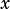-axis at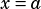and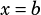, what is the value of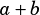?

A)
B)
C)
D)